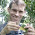## Thursday, October 11, 2012

### Ancestral character estimation under the threshold model

Since posting about it on the anoleannals.org blog, I have been working on a first pass at ancestral character estimation under the threshold model.

Under the threshold model, the phenotypic trait of interest has a discretely valued presentation - for instance, color or habitat*; but a continuous, unmeasurable, underlying liability. (*Note, of course, that a characteristic like habitat might be judged by measuring a number of quantitative features about the environment - this is not a serious problem.) When the liability exceeds some threshold value, the discretely valued state of the observable character trait changes. So, for instance, the figure below (which I already used in a previous post) shows evolution of liability on a phylogenetic tree. When the liability crosses a threshold, the observable trait (here shown as a set of colors) changes state. For more details on the threshold model, which derives originally from quantitative genetics, I would encourage you to check out Felsenstein (2012) and/or my prior posts on the subject (1, 2, 3).

The model provides several advantages. One is that it may be inherently more realistic than the discrete Markov model that we typically use for discretely-valued character traits. This model is the same as the "nucleotide model" that we use for DNA sequence evolution in phylogeny inference, and thus may not be well suited to complex traits typical of organismal phenotypes.

OK, so in principle, applying the threshold model to ancestral character estimation shouldn't be that different from applying it other problems. If we are to use Bayesian MCMC (as I have done in this case), we should just sample the liabilities for the tips of the tree and internal nodes; compute their probability under our model for evolution of the liabilities; and then multiply this probability by 1.0 if the liabilities predict our observed phenotypes, and 0.0 otherwise. Since it is not too interesting to reconstruct a trait that only has a binary presentation, I have also added MCMC sampling of the "positions" of the thresholds. (Our liabilities are unobserved, and thus scaleless, so these positions can only be interpreted in relative terms.)

Unfortunately, I have to run - so I will post more about this later, but below I've plotted the results from a single MCMC run of this method. The colored circles at the tips are the tip states for the discrete character, and the pie graphs at internal nodes represent the posterior probability of the internal nodes between in each of the four observed states, under the threshold model.

> source("ancThresh.R")
> tree<-pbtree(n=100)
> l<-fastBM(tree,internal=TRUE) # simulate liability
> # discretize!
> x<-rep("blue",length(l))
> x[l>0]<-"green"
> x[l>1]<-"red"
> x[l>2]<-"yellow"
> names(x)<-names(l)
> # run the MCMC
> result<-ancThresh(tree,x[1:length(tree\$tip)],ngen=100000, control=list(sample=1000))
**** NOTE: no sequence provided, using alphabetical or numerical order
 "gen 1000"
 "gen 2000"
...

Pretty cool! I will post a lot more on this very soon.

1.This is pretty awesome. I'm still wrapping my head around these concepts, so I'll just leave a quick question here. In the figure and the simulation, it appears to me that in the case of a discrete trait with more than 2 state, they have to be (or assumed to be) ordered in relation to the liability, am I correct? i.e. it's easier to get to "yellow" from "red" than from "green".

Is this interpretation right? if so, would there be a way around this? would something like a "multivariate liability" with n-1 orthogonal axes work (n being the number of discrete states? Could some sort of test of sequential (or stepwise) evolution be done by comparing these models, or modifying the liability covariances?

Anyways, I know this might not be necessarily something interesting, just trying to link these concepts to the empirical problems. This approach seems really promising. Thanks!

1.This is absolutely correct, and a direct consequence of the model. I will discuss this (among other things) in a longer post to come.

One possibility would be to use information theoretic criteria (for instance) to compare among alternate orderings for the discrete character along the liability axis.

This is literally on the bleeding edge of what I'm working on here - so I haven't tested this at all yet.

Note: due to the very large amount of spam, all comments are now automatically submitted for moderation.